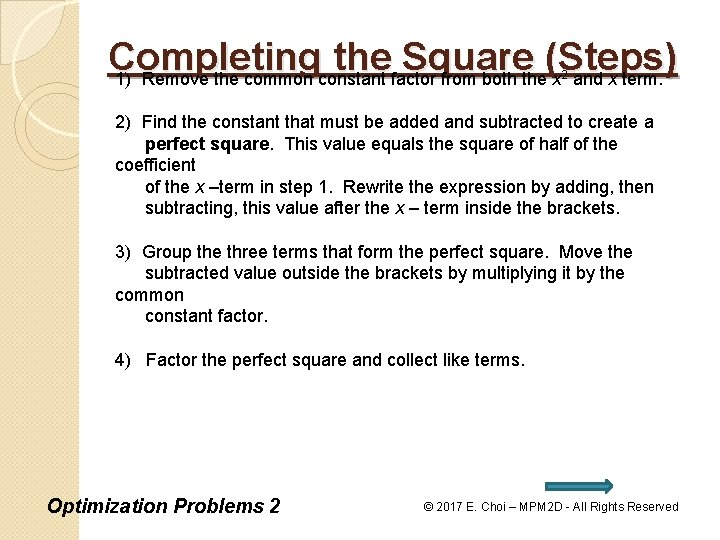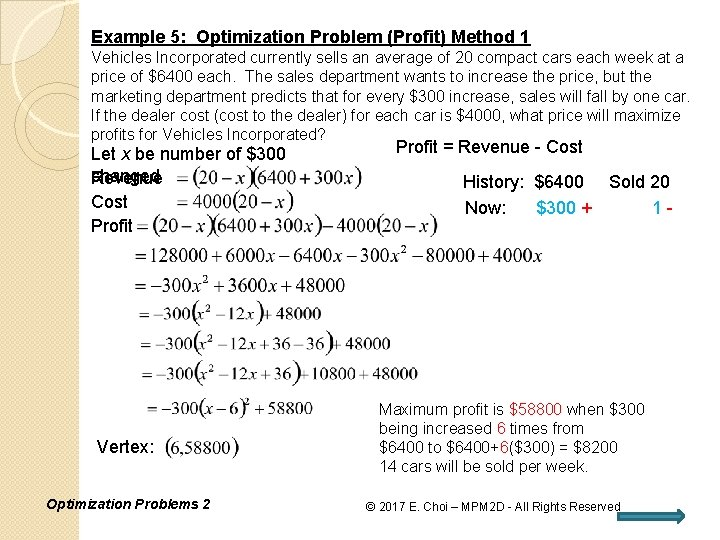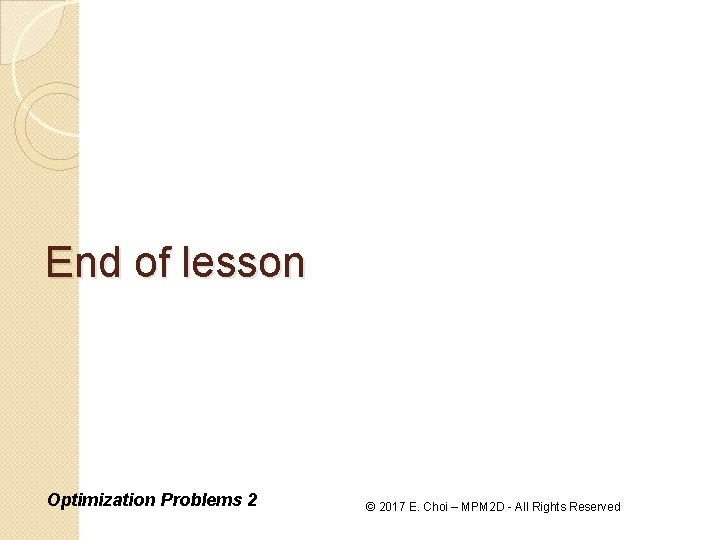• Slides: 9Completing the Square • A quadratic relation in standard form can be rewritten in vertex form by creating a perfect square in the expression, then factoring the square. This technique is called completing the square. • Completing the square can be used to find the vertex of a quadratic in standard form without finding the zeros of the relation or two points equidistant from the axis of summetry. • Completing the square allows you to find the maximum or minimum value of a quadratic relation algebraically, without using a graph. Optimization Problems 2 © 2017 E. Choi – MPM 2 D - All Rights ReservedCompleting the Square (Steps) 1) Remove the common constant factor from both the x and x term. 2 2) Find the constant that must be added and subtracted to create a perfect square. This value equals the square of half of the coefficient of the x –term in step 1. Rewrite the expression by adding, then subtracting, this value after the x – term inside the brackets. 3) Group the three terms that form the perfect square. Move the subtracted value outside the brackets by multiplying it by the common constant factor. 4) Factor the perfect square and collect like terms. Optimization Problems 2 © 2017 E. Choi – MPM 2 D - All Rights ReservedSummary: Quadratic Formulas Vertex Form: Vertex: Axis of Symmetry: x-intercepts: y-intercept: Standard Form: Vertex: Axis of Symmetry: x-intercepts: y-intercept: Optimization Problems 2 © 2017 E. Choi – MPM 2 D - All Rights ReservedExample 4: Optimization Problem (Area) An enclosure is constructed with the shape shown and with a perimeter of 600 m. What are the values of x and y so that the area of the enclosure is a. There maximum? are missing sides in the shape!! 3 x Given: x+y M 2: By completing the square: Square + Rectangle M 1: By Factoring: Roots: Axis of symmetry: Optimization Problems 2 Vertex: Objective Relation Standard form!! Opens down, max value occurs! Vertex: Therefore maximum area is 360000/19 m 2 (18947. 4 m 2) when x is 600/19 m (31. 6 m) and y is 142. 1 m. © 2017 E. Choi – MPM 2 D - All Rights ReservedExample 5: Optimization Problem (Profit) Method 1 Vehicles Incorporated currently sells an average of 20 compact cars each week at a price of \$6400 each. The sales department wants to increase the price, but the marketing department predicts that for every \$300 increase, sales will fall by one car. If the dealer cost (cost to the dealer) for each car is \$4000, what price will maximize profits for Vehicles Incorporated? Let x be number of \$300 changed Revenue Cost Profit Vertex: Optimization Problems 2 Profit = Revenue - Cost History: \$6400 Sold 20 Now: \$300 + 1 - Maximum profit is \$58800 when \$300 being increased 6 times from \$6400 to \$6400+6(\$300) = \$8200 14 cars will be sold per week. © 2017 E. Choi – MPM 2 D - All Rights ReservedExample 5: Optimization Problem (Profit) Method 2 Vehicles Incorporated currently sells an average of 20 compact cars each week at a price of \$6400 each. The sales department wants to increase the price, but the marketing department predicts that for every \$300 increase, sales will fall by one car. If the dealer cost (cost to the dealer) for each car is \$4000, what price will maximize profits for Vehicles Incorporated? Let x be number of \$300 changed Revenue Cost Profit = Revenue - Cost Maximum profit is \$58800 when \$300 being increased 6 times from \$6400 to \$6400+6(\$300) = \$8200 14 cars will be sold per week. Vertex: Optimization Problems 2 © 2017 E. Choi – MPM 2 D - All Rights ReservedHomework Work sheet: Optimization Problems #11 - 16 Text: P. 355 #21, 22 P. 391 #17, 18, 20, 23 Check the website for updates Optimization Problems 2 © 2017 E. Choi – MPM 2 D - All Rights Reserved